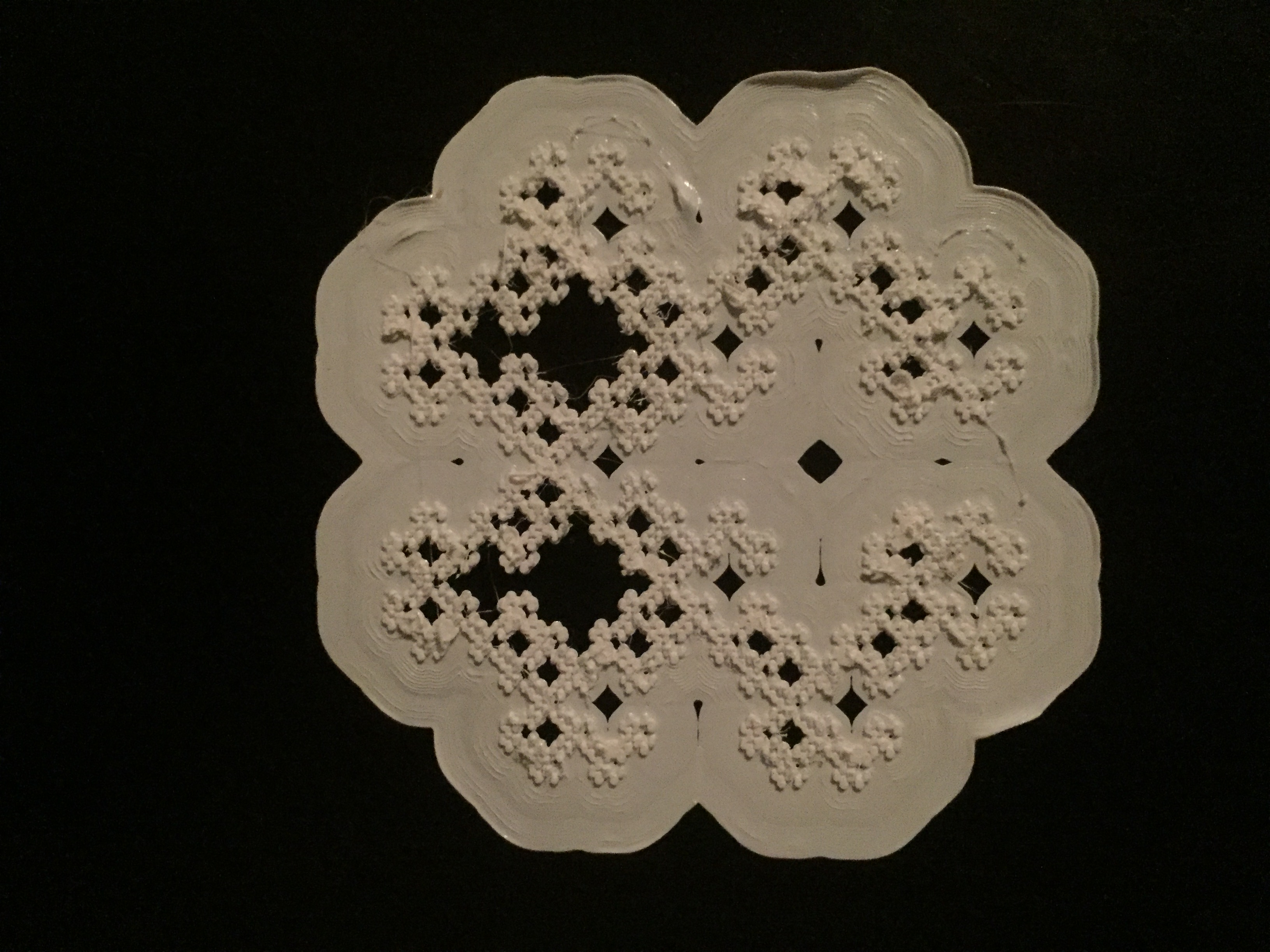# Supreme Fractal Carpet

This is my fractal carpet. The total surface area is 4*4 that is 16, I remove 5 pieces of the total area  that become 16-5=11

The following pictures is my different level of Fractal Carpet:

Level 1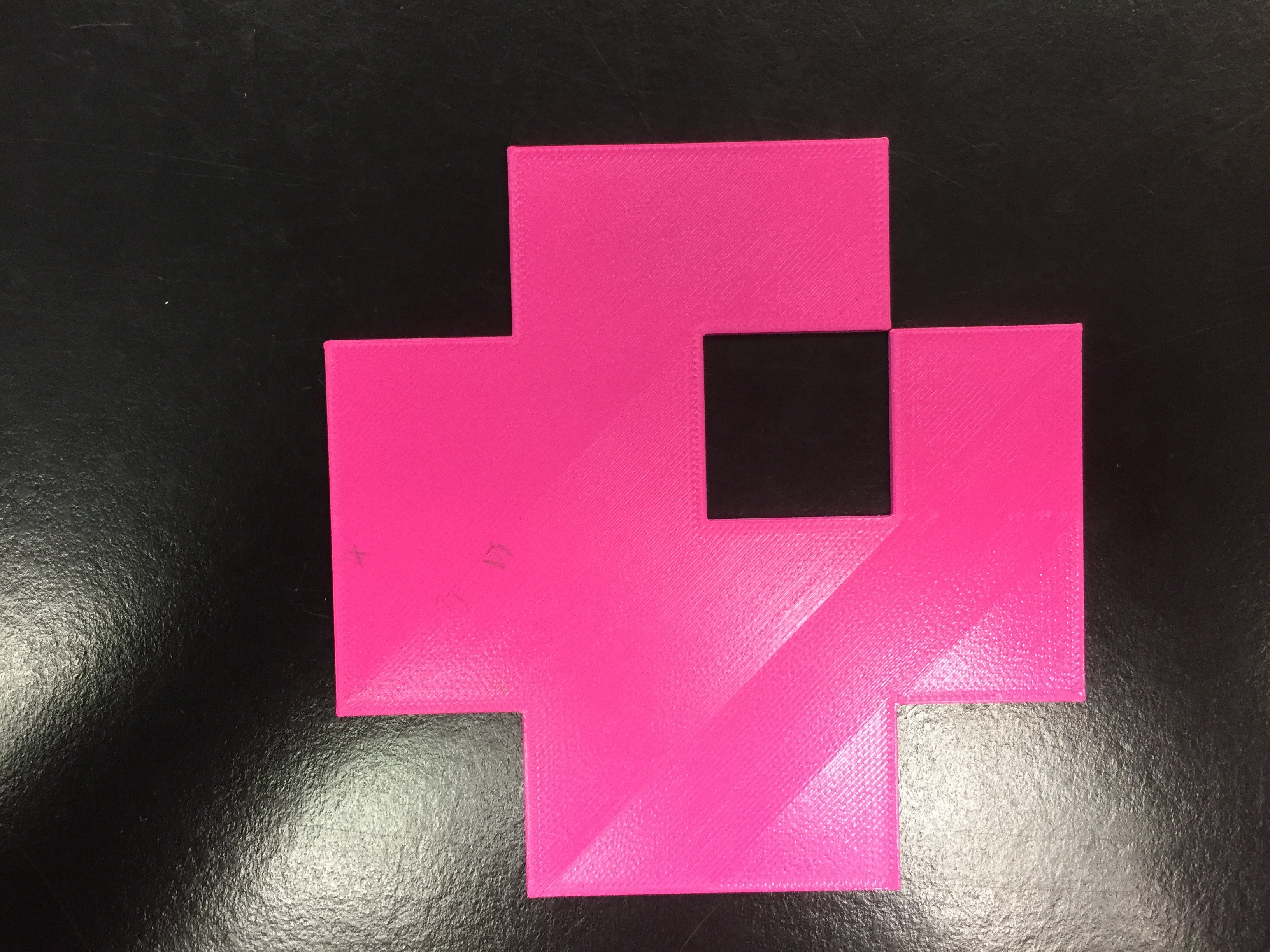Level 2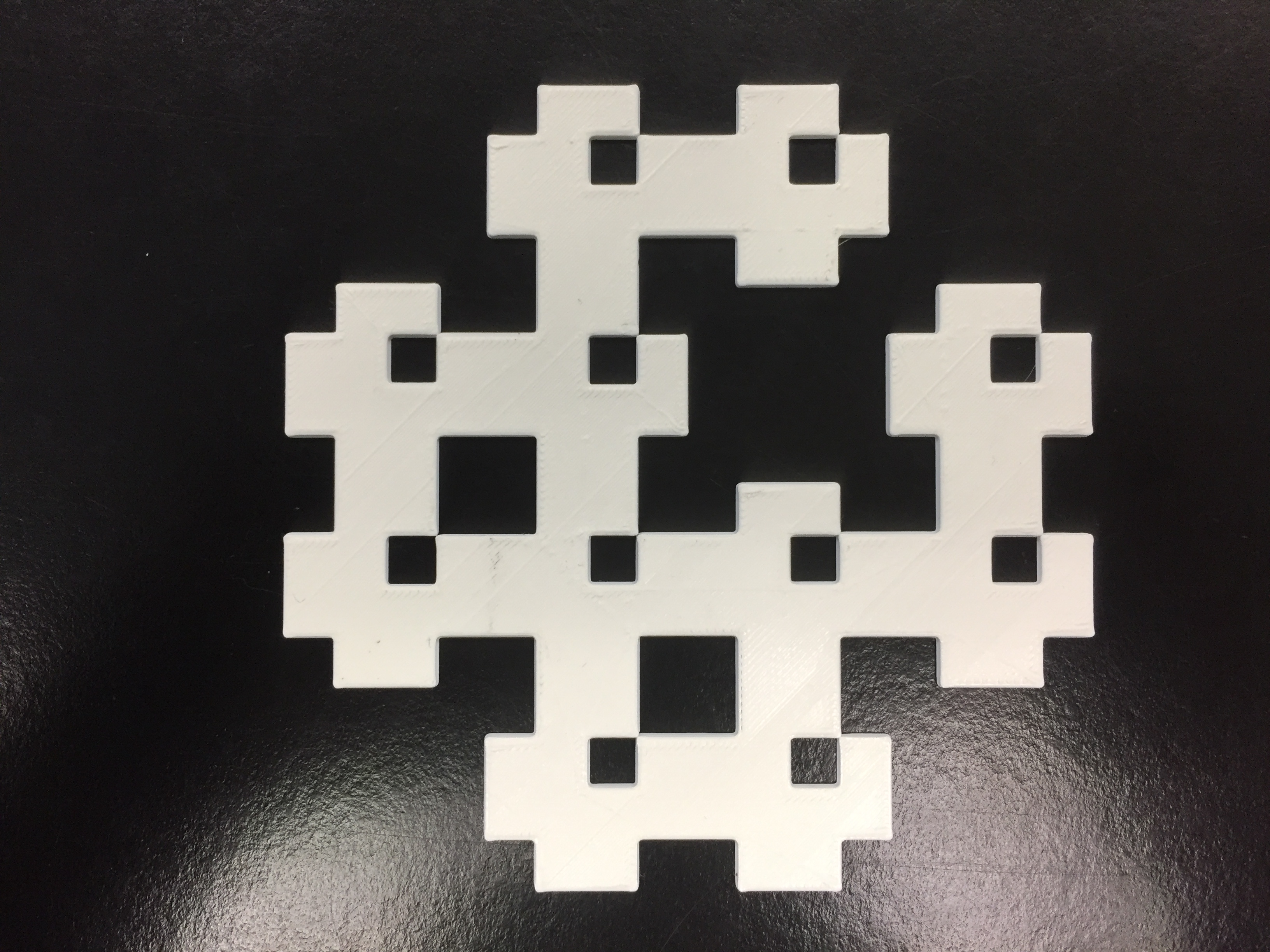Level 3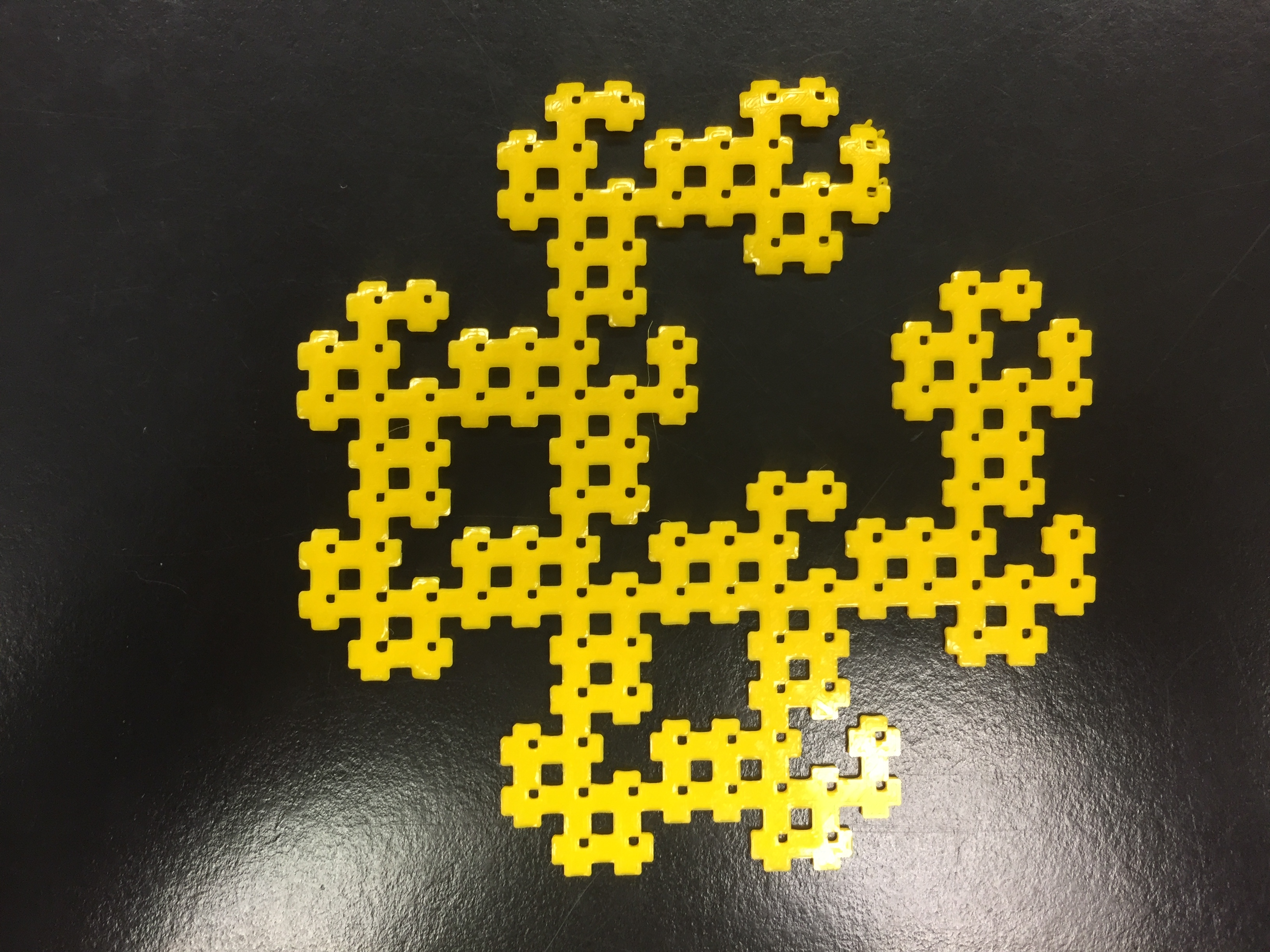This is the compare between the total area and my Level 1 fractal carpet.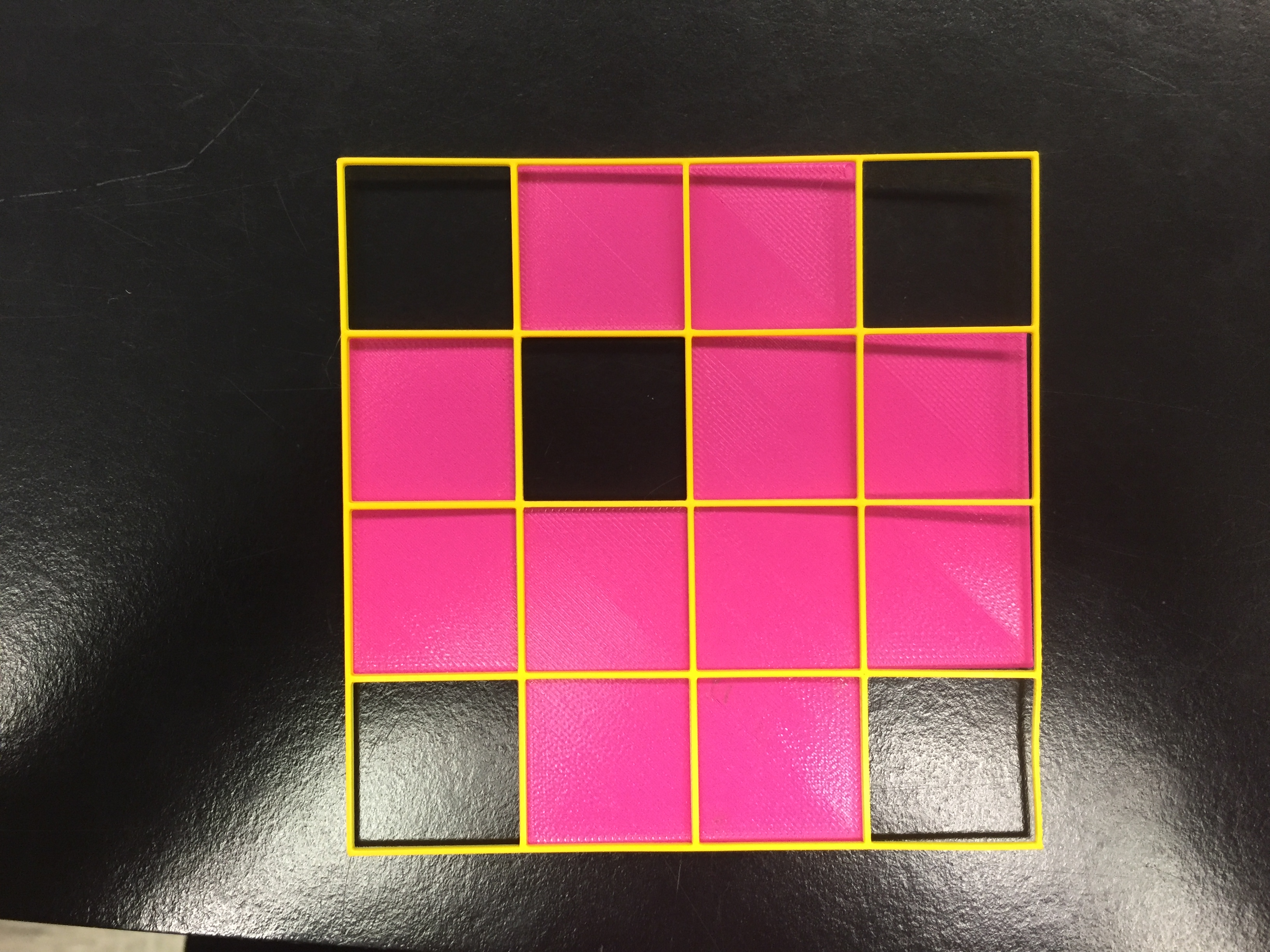This is the compare between the total area and my Level 3 fractal carpet.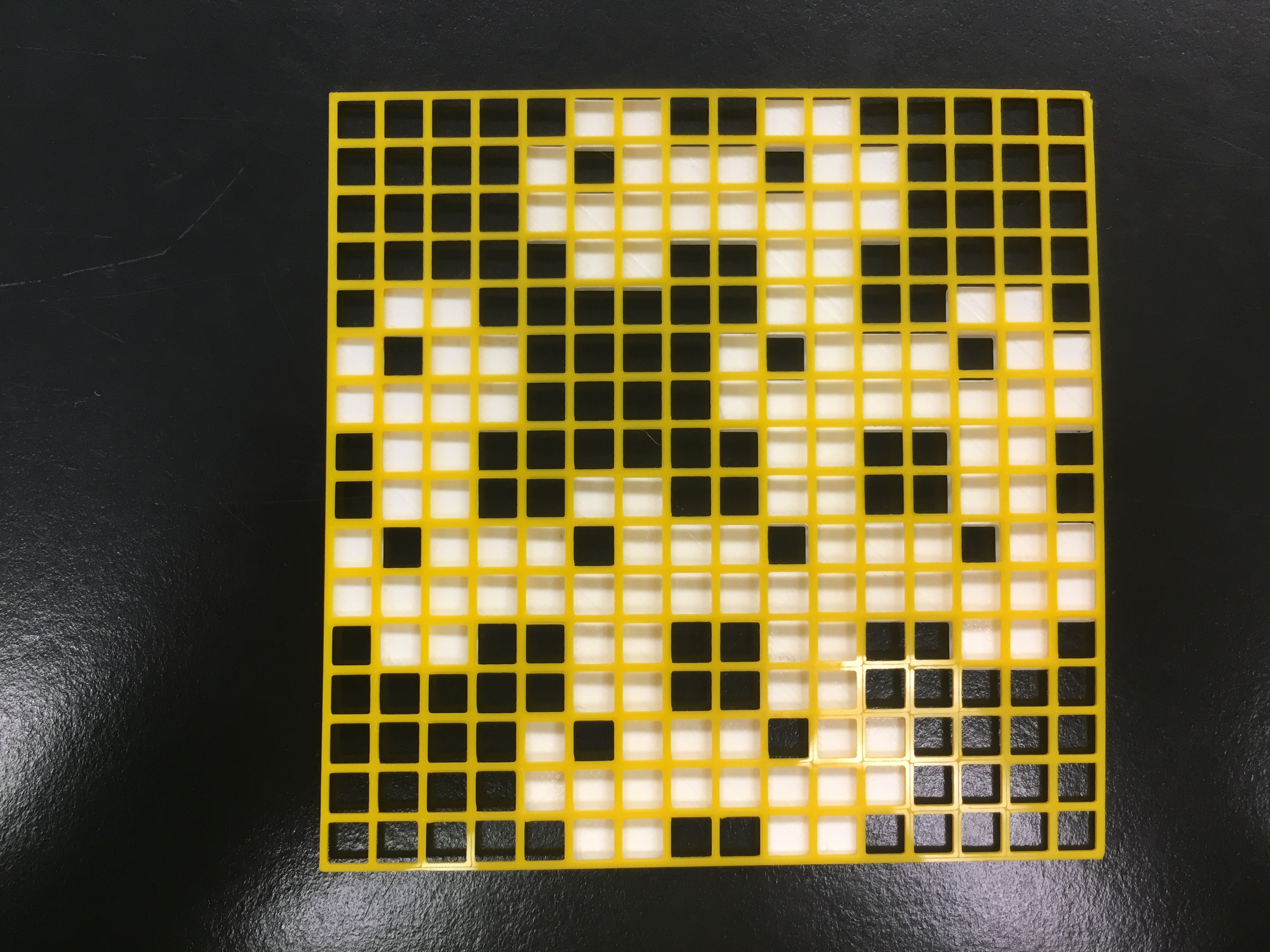### First Calculation of Area:

I calculate area for this fractal as the following step:

###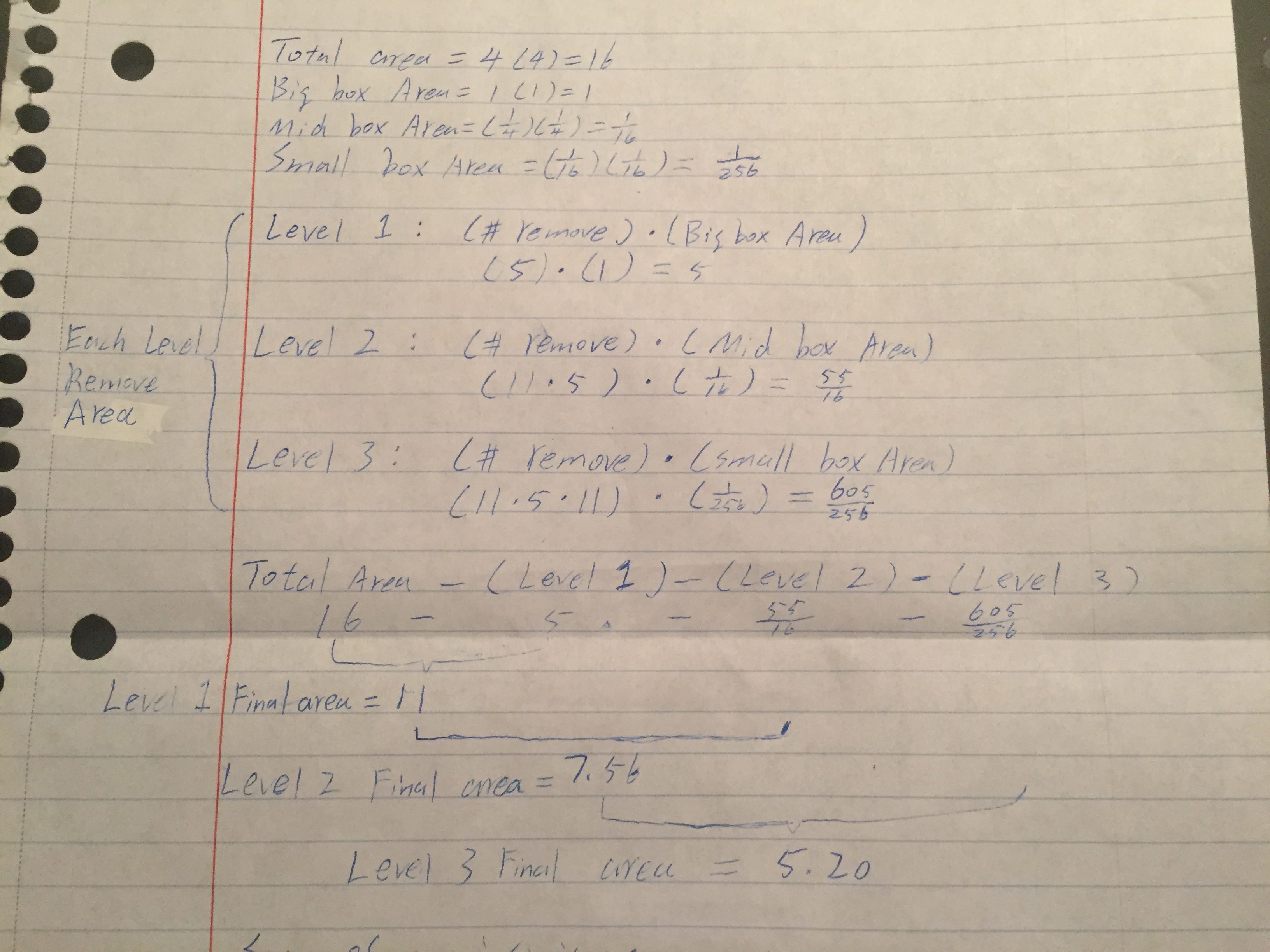The first step is know the area of each level box areas.

Total area=4*4=16

Big box area=1*1=1

Mid box area=(1/4)*(1/4)

Small box area=(1/16)*(1/16)

The second step is calculate the remove area of each level.

Level 1 : (number of remove)*(Big box area) =5*1=5

Level 2 : (number of remove)*(Mid box area) =(11*5)*(1/16)=55/16

Level 3 : (number of remove)*(Small box area) =(11*5*11)*(1/256)=605/256

The final step is use the total area divide the remove area of the Level 1,2,3.

Total area – (Level 1)- (Level 2)- (Level 3)

16-(5)-(55/16)-(605/256)

Level 1 Final area is 11

Level 2 Final area is 7.56

Level 3 Final area is 5.20

### Geometric SeriesCalculations: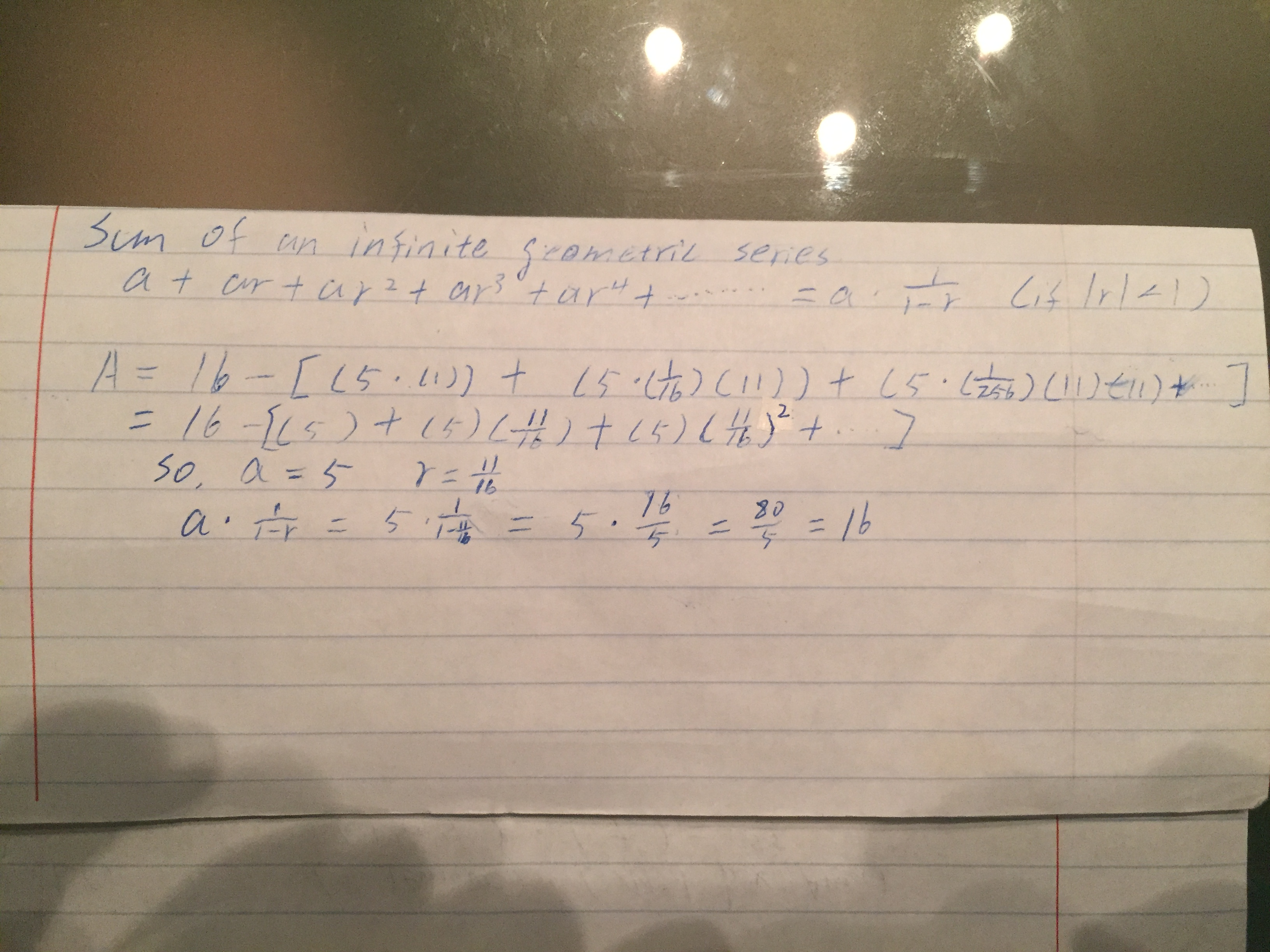This calculate is the find the Fractal Carpet is infinite or finite. When we calculate the geometric series we need to know three things that are x, a and r. The x is initial area, r is the first term and a is the common ratio. We used the common ratio to multiply by different root of r and plus them together. Finally, we can sum the formula is a multiply by 1 divide by 1-r. When we used the x minus the formula and also plug the number on the formula we can get the process like the picture.  According to the above calculation I got the answer is 0 and the a is 5, r is 11/16. because the r is small then 1, this Fractal Carpet should be finite.

Dimension Calculations:

When we calculated the dimension of Fractal Carpet we need to know each size of different levels. The second level’s area is 16 because it is consist of 16 pieces same side square. The next level of the area is divided by 4 of first pieces. It can be show like 1/4 of each in the the second level’s pieces. My Fractal Carpet is remove 5 pieces from the second level so the area will be 1/11. The formula is A^D=B and the A is 1/4, B is 1/11. When we put the number on the formula it will be:

（1/4）d = 1/11

4 ^ d = 11

log（4，11）= D

D=1.7297

# Sharing on the Thingiverse

https://www.thingiverse.com/thing:2675926

# level 4